## Example Questions

1 3 Next →

### Example Question #42 : Proportion / Ratio / Rate

Mary travels at a constant speed to her friend Clara's house by walking directly east for 8 miles, and then walking directly north for 6 miles. The amount of time that it takes her to reach Clara's house is equal to t. If Mary had been able to travel directly to Clara's house in a straight line at the same speed, how much time would she have saved, in terms of t?

4t/7

2t/7

6t/7

3t/7

5t/7

2t/7

Explanation: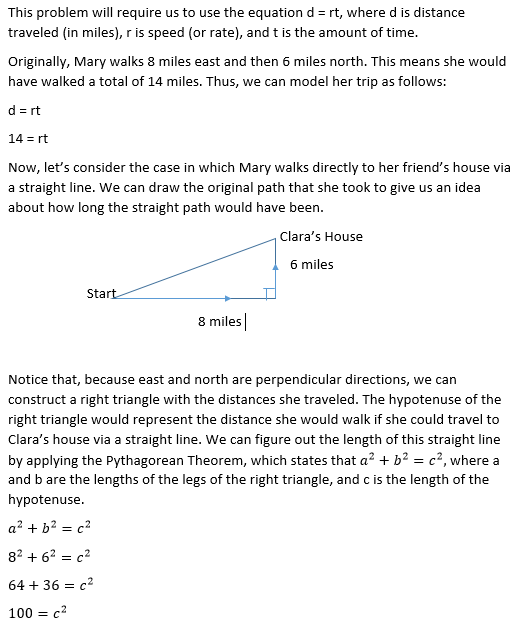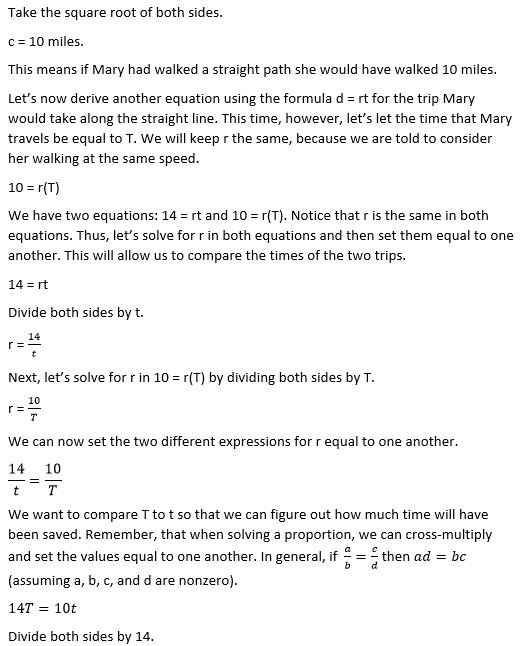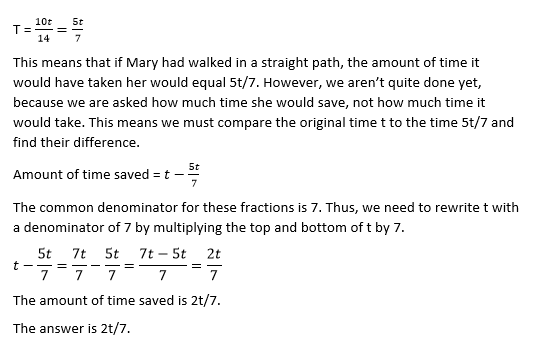### Example Question #43 : Proportion / Ratio / Rate

Sam earns d dollars per day. At this rate, how many days will it take Sam to earn q dollars?

q/d

d/q

100d/q

dq

10q

q/d

Explanation:

q is the number of dollars to be earned, d is the amount earned each day, so you divide q by d to get the number of days.

### Example Question #44 : Proportion / Ratio / Rate

Bob can build a house in 3 days. Gary can build a house in 5 days. How long does it take them to build a house together?

5/4 days

4 days

3/2 days

15/8 days

2 days

15/8 days

Explanation:

DO NOT pick 4 days, which would be the middle number between Bob and Gary's rates of 3 and 5 days respectively. The middle rate is the answer that students always want to pick, so the SAT will provide it as an answer to trick you!

Let's think about this intuitively before we actually solve it, so hopefully you won't be tempted to pick a trick answer ever again! Bob can build the house in 3 days if he works by himself, so with someone else helping him, it has to take less than 3 days to build the house! This will always be true. Never pick the middle rate on a combined rates problem like this!

Now let's look at the problem computationally. Bob can build a house in 3 days, so he builds 1/3 of a house in 1 day. Similarly, Gary can build a house in 5 days, so he builds 1/5 of a house in 1 day. Then together they build 1/3 + 1/5 = 5/15 + 3/15 = 8/15 of the house in 1 day.

Now, just as we did to see how much house Gary and Bob can build separately in one day, we can take the reciprocal of 8/15 to see how many days it takes them to build a house together. (When we took the reciprocal for Bob, 3 days/1 house = 1/3 house per day.) The reciprocal of 8/15 is 15/8, so they took 15/8 days to build the house together. 15/8 days is almost 2 days, which seems like a reasonable answer. Make sure your answer choices make sense when you are solving word problems!

### Example Question #45 : Proportion / Ratio / Rate

Two brothers, Jake and Fred, have a pool in which 9 laps is 1 mile. Jake swims 2 laps in 1 minute, and Fred swims 4 laps in 1 minute. How far has Jake swum when Fred has finished swimming 2 miles?

3/4 miles

2 miles

1 mile

9/2 miles

3/2 miles

1 mile

Explanation:

We can solve this with lots of calculations and conversions of laps to miles, etc., or we can look at what the question is really asking. We want to know how far Jake swims in the time it takes Fred to swim two miles. Instead of converting Fred's miles to laps and comparing to Jake's laps, let's just look at how fast the two brothers swim in relation to one another. Fred swims twice as fast as Jake, so in the same amount of time, he will swim twice as far as Jake. Therefore if Fred swims 2 miles, Jake swims 1 mile in the same amount of time.

### Example Question #46 : Proportion / Ratio / Rate

Every 3 minutes, 4 liters of water are poured into a 2,000-liter tank. After 4 hours, what percent of the tank is full?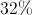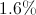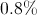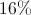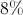Explanation:

60 minutes in an hour, 240 minutes in four hours. If 4 liters are poured every 3 minutes, then 4 liters are poured 80 times. That comes out to 320 liters. The tank holds 2,000 liters, so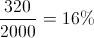of the tank is full.

### Example Question #41 : Proportion / Ratio / Rate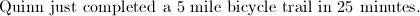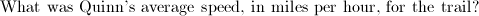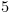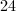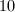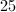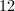Explanation: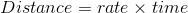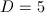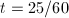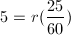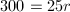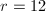### Example Question #48 : Proportion / Ratio / Rate

Laura owns a large property. Her lawn is rectangular. It is 500 meters long, and 350 meters wide. If Laura mows the lawn at a rate of 20,000 meters squared per hour, how many hours will it take Laura to finish mowing the lawn?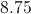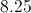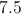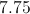Explanation:

The area of a rectangle is the length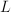, multiplied by the width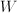. Here the area of the lawn in meters squared is: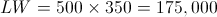We found that Laura is mowing 175,000 meters squared at a rate of 20,000 meters squared per hour.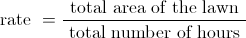Plugging in 20,000 for the rate, and 175,000 for the total area gives: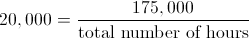Multiply both sides by the total number of hours: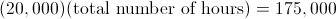Now, divide both sides by 20,000: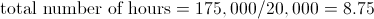### Example Question #51 : Proportion / Ratio / Rate

Jess is trying to fill her 10,000 gallon pool with water before the summer. She has three hoses, one that pump water at a rate of 175 gallons per hour, 25 gallons per hour and 200 gallons per hour. If she used all three hoses how many hours would it take to fill her pool?Explanation:

First add up all of the rates to get the total rate of water flowing into the pool at one time.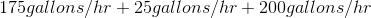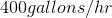Then to determine the time it takes to fill the pool divide the total volume of the pool by this rate.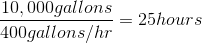### Example Question #52 : Proportion / Ratio / Rate

Joaquin can clean a pool in j minutes.  River can clean the same size pool in r minutes.  Which of the following expresses the time needed for three pools (all of the same size) to be cleaned when Joaquin and River work together?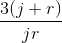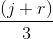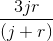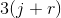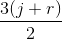Explanation:

This is a rate question, so first we should rewrite the "times" given to us as rates.

Joaquin's rate :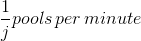River's rate: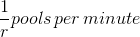Now we add their rates together, to find the rate when they work together: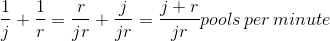Using the equiation for distance, d = rate * time we can rearrange it to see that to find time we need to do t = distance/rate

in this case, the distance is "3 pools" and the rate is "(j+r)/jr pools per minute".  So we reach our answer:### Example Question #39 : Proportion / Ratio / Rate

If Kara drives a distance of miles every hours, how many hours will it take her to drive a distance of miles, in terms of m, h, and d ?

hmd

dhm

dhm

dmh

mhd